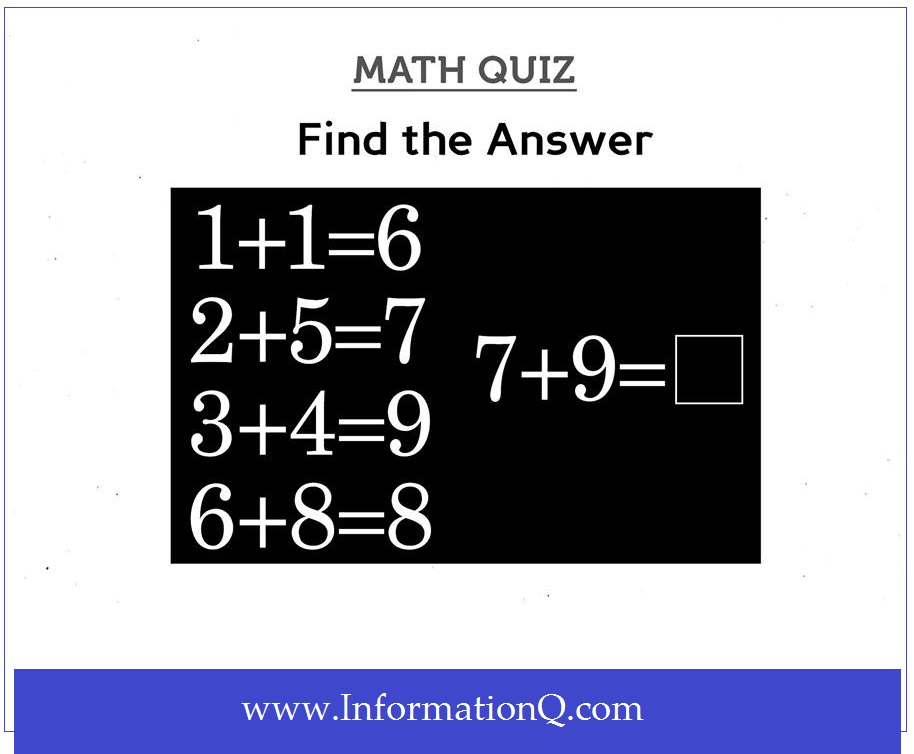# Simple Math Quiz Questions for Kids

Question:

If 1+1=6
2+5=7
3+4=9
6+8=8
Then 7+9=?Here
2+5=7
& 3+4=9
Then 7+9 must be equal to sum of (2+5) & (3+4)

as (2+5)=7 & (3+4)=9
Thus,
7+9=2+5+3+4=14